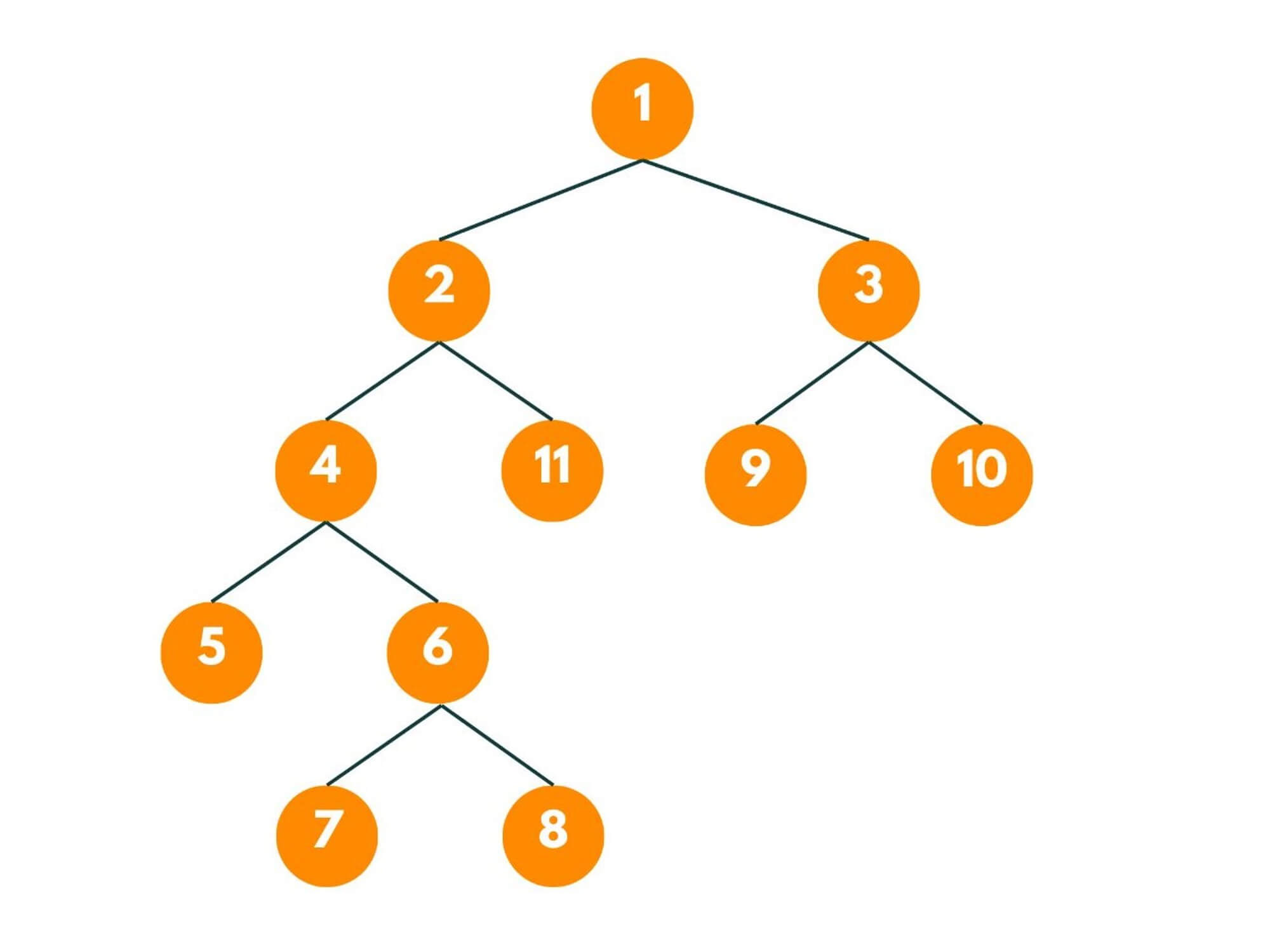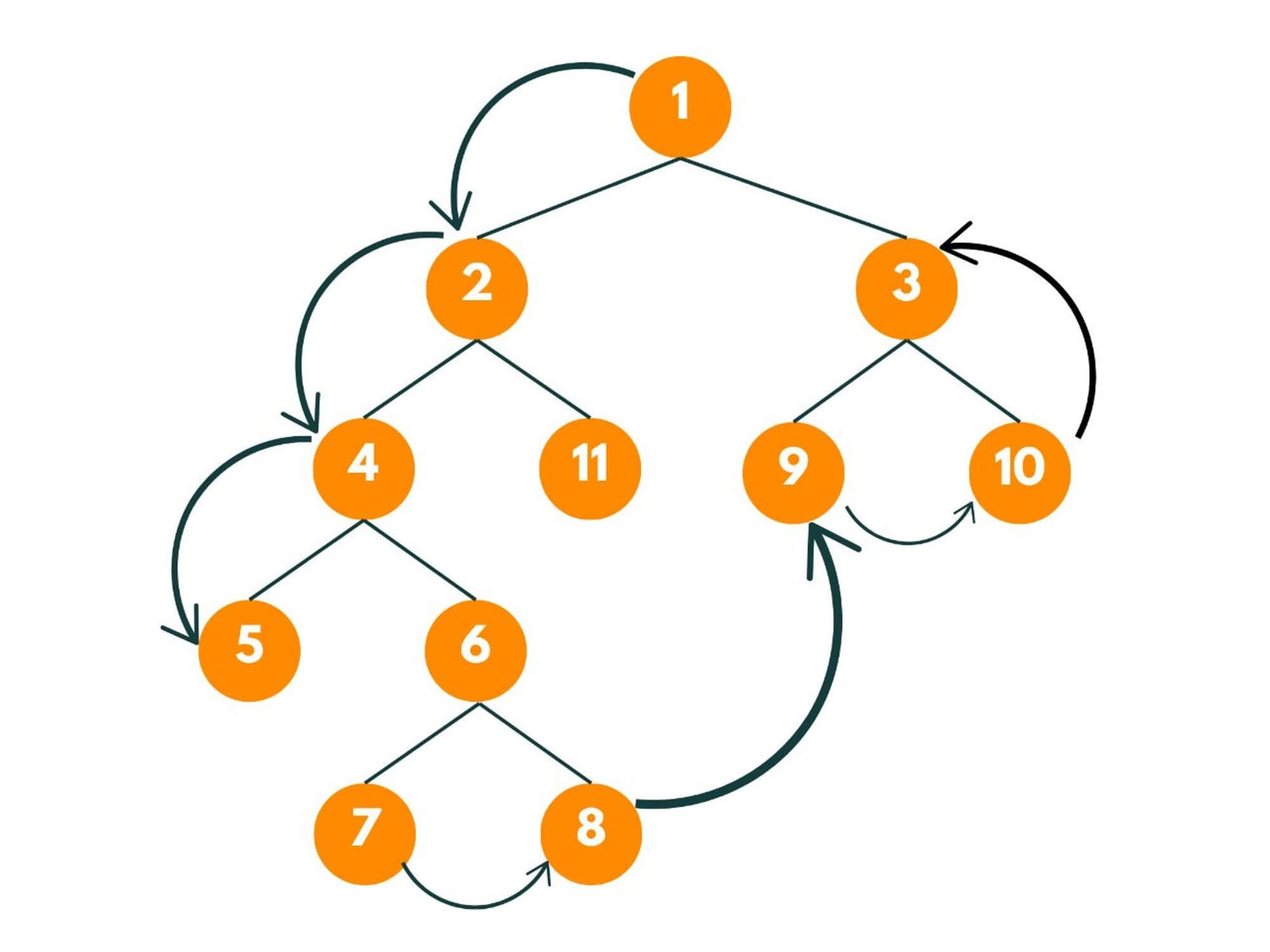# Boundary Traversal of Binary Tree (with code)

Binary trees are fundamental data structures used in computer science and have various applications in the real world. In this article, we will delve into the concept of boundary traversal of a binary tree and explore different approaches to solve this problem efficiently.

## Boundary Traversal of Binary Tree

Traversing a binary tree refers to visiting each node in a specific order. One common traversal problem is boundary traversal, which aims to explore the perimeter of a binary tree, including its left boundary, leaves, and right boundary.

Here is the problem statement: "We have been given a binary tree and we need to print the boundary nodes of the binary tree. First, we need to print the root node and the left nodes followed by printing the left nodes, and last, the right nodes are to be printed in reverse order."

Let's understand the problem statement with an example:So first we print the root node i.e. 1, then the left nodes - 2, 4, then the leaf nodes- 5, 7, 8, 9, 10 followed by right nodes - 3. So the output will be 1, 2, 4, 5, 7, 8, 9, 10, 3.So we will be given the root node of the binary tree and a function boundaryTraversal where we need to return all the nodes arranged n the given order in the form of an ArrayList. The basic idea here will be to create 3 functions:

• Function A, which would print the left nodes.
• Function B, which would print the leaf nodes.
• Function C, which would print the right nodes in reverse order.

### Solution for Binary Tree Boundary Traversal

Here is a step-by-step algorithm for boundary traversal of a binary tree:

1. We check the basic condition that if the given root node is null, we return an empty ArrayList.
2. If the root is not null, we first add the root data to the resultant ArrayList.
3. We will create a boolean function isLeafNode() which would return true if the given node is a leaf node or not i.e., the right node and left node of the given node is null.
4. Now we create a function addLeftNode() which would add the left nodes to the ArrayList. We will pass the root node and resultant ArrayList as arguments in this function.
1. Now we create the current node pointing to the root node.
2. Now if the curr node is not a leaf node, we add the node data to the resultant ArrayList.
3. Then we point the current node to the next left node.
4. We repeat these steps till the current node is not equal to null.
5. Now we create a function addRightNode() which would add the right nodes to the ArrayList in reverse order. We will pass the root node and resultant ArrayList as arguments in this function.
1. First, we create an ArrayList of Integers say, temp and well will add data of all right nodes to this ArrayList.
2. Now if the curr node is not a leaf node, we add the node data to the resultant ArrayList.
3. Then we point the current node to the next right node.
4. We repeat these steps till the current node is not equal to null.
5. At the end, we reverse the temp ArrayList and add it to the resultant ArrayList.
6. At last, we create a function addLeafNode() that would add all the leaf nodes to the resultant ArrayList.
1. First, we check if the current node is a leaf node or not.
2. While the current node is not equal to null, we recursively call the addLeafNode() and pass the left and right nodes of the current node as arguments.
7. In the main function, we call the addLeftNode() then addRightNode() and addLeafNode() respectively, and return the resultant ArrayList.

Here is the simple implementation for boundary traversal in a binary tree:

```// class Node
// {
//     int data;
//     Node left, right;

//     public Node(int d)
//     {
//         data = d;
//         left = right = null;
//     }
// }

class Solution{

ArrayList <Integer> boundary(Node root){

ArrayList<Integer> res = new ArrayList<>();

//checking is root node is not equal to null.
if(root == null) return res;

//adding data of root node to res.

//returning the result
return res;
}

boolean isLeafNode(Node root){
return root.left == null && root.right == null;
}

//checking if given node is leaf node or not.
if(isLeafNode(root)){
return;
}
// passing the left child of root to this function.

// passing the right child of root to this function.
}

//current point to the root node.
Node current = root;

while(current != null){
//checking if given node is leaf or not.

// if child is not null, current will point to its child.
if(current.left != null) current = current.left;
else current = current.right;
}
}

//current point to the root node.
Node current = root;

ArrayList<Integer> temp = new ArrayList<>();

while(current != null){
//checking if given node is leaf or not.

// if child is not null, current will point to its child.
if(current.right != null) current = current.right;
else current = current.left;
}

Collections.reverse(temp);
for(int i : temp){
}
}
}
```

### Time & Space Complexity

The time complexity of the boundary traversal algorithm is O(n), where n is the number of nodes in the binary tree. This is because we need to visit each node once to perform the traversal.

The space complexity of the algorithm is O(h), where h is the height of the binary tree. This is because the space used by the recursive function calls and the auxiliary stack in the iterative part depends on the height of the tree. In the worst case, where the binary tree is skewed and has a height of n, the space complexity becomes O(n).

However, in balanced binary trees, the height is typically log(n), resulting in a space complexity of O(log(n)).

## Conclusion

The boundary traversal of a binary tree allows us to explore and collect the nodes lying on its perimeter. By understanding the concept of boundary traversal and implementing the appropriate approach, you can easily solve this problem and gain valuable insights into the structure of it.

### FavTutor - 24x7 Live Coding Help from Expert Tutors!